Inline mesh generation and adaptation based on Triangle

In this document we demonstrate how to generate unstructured 2D meshes for oomph-lib, using Jonathan Shewchuk's Triangle library from within an oomph-lib driver code. This complements the discussion in another tutorial where we illustrated how to build oomph-lib meshes using the output generated when Triangle is used to create the mesh externally.

• how to create meshes with polygonal or curvilinear boundaries

and

• how to adapt (re-generate) these meshes based on spatial error estimates.

Here we restrict ourselves to the solution of problems in fixed domains. Other tutorials discuss more advanced applications involving moving meshes, e.g.

# Overview of mesh generation procedures

## Meshes with polygonal boundaries

If the domain has polygonal outer (and possibly internal) boundaries, the mesh generation process follows a similar pattern to that required in the external use of Triangle , but using oomph-lib classes to represent the required data. We define the polygonal boundaries using TriangleMeshPolyLines, which represent distinct mesh boundaries and are defined by a series of vertex coordinates. Multiple TriangleMeshPolyLines can be combined to define (closed) TriangleMeshPolygons. These are then used to create an unstructured mesh with a pre-determined target area for all elements, via an interface to Triangle .

One particular feature of oomph-lib's interface to Triangle is that each closed TriangleMeshPolygon must contain at least two distinct TriangleMeshPolyLines, each with its own boundary ID. This is because every Node can only have a single-valued boundary coordinate but if the boundary is closed there would be a discontinuity in the coordinate value. (For example, a standard representation would have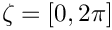, but the node at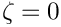should also have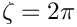and this is not possible.) The sketch below shows a representative domain as well as two legal and one illegal representations of the domain boundaries. Note that the boundaries can be enumerated in an arbitrary fashion.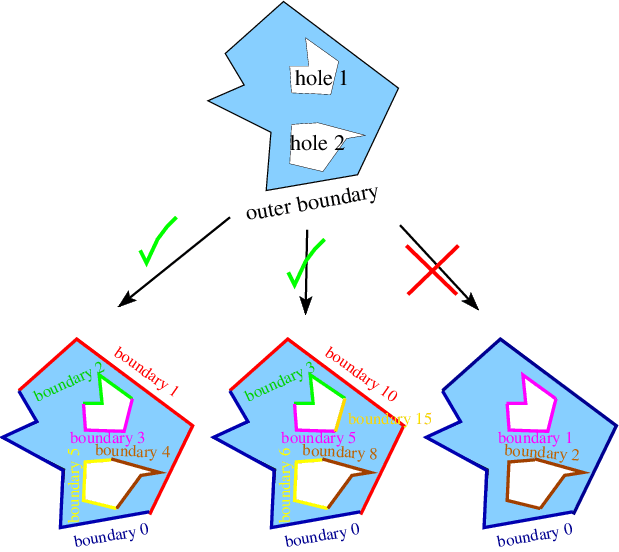Sketch of a polygonal domain (top) and two legal (bottom left and middle) and one illegal (bottom right) representations of the boundaries in terms of TriangleMeshPolyLines.

## Meshes with curvilinear boundaries

It is also possible to discretise domains with curvilinear boundaries as shown in the sketch below. Assuming that each curvilinear boundary is represented by a GeomObject that specifies the position vector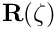to a point on the curvilinear boundary as a function of some surface coordinate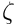, we split each closed boundary into (at least) two distinct TriangleMeshCurviLines – the curvilinear equivalents of TriangleMeshPolyLines. Each TriangleMeshCurviLine is constructed from a pointer to the GeomObject and the start and end values of the boundary coordinatealong the relevant part of the curvilinear boundary. The TriangleMeshCurviLines are then combined to a TriangleMeshClosedCurve – the general closed curve.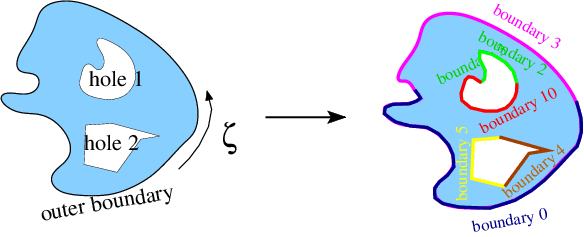Sketch of a domain bounded by a curvilinear boundary, containing two holes with curvilinear (hole 1) and polygonal (hole 2) boundaries, respectively.

The mesh is then created in a two-stage process: All TriangleMeshCurviLines are sampled at a certain number of points (specified by the user in the constructor) to create the vertices for a polyline representation of the boundary. This polygonal representation of the boundaries is used by Triangle to generate the mesh. Finally, nodes on curvilinear boundaries are "snapped" onto the actual curvilinear boundary.

# Overview of mesh adaptation methodology

The methodology employed to adapt oomph-lib's unstructured meshes differs from that used for structured meshes. Specifically, rather than sub-dividing elements in which the error estimate exceeds a threshold and merging elements in which the solution is "too accurate", we completely re-generate the mesh and project the solution from the old to the new mesh. This is because we originally developed the underlying methodology to solve free-boundary problems in which the domain undergoes such large deformations that re-meshing is required. The ability to adjust the element sizes guided by spatial error estimates when re-meshing the domain is a simple fringe benefit.

A number of issues are important:

• To facilitate mesh adaptation in free-boundary problems, we re-generate the polygonal representation of the boundary, using the vertex nodes of the elements on the boundary to re-define the polygon. The number of vertices in the polygons that define the mesh boundaries will therefore generally change during the mesh adaptation process. This is discussed in more detail in another tutorial.

• The mesh (and thus its constituent elements) are completely re-generated when the mesh is adapted, and so it is necessary to "complete the build" of all elements after each mesh adaptation. For instance, pointers to problem parameters (Reynolds numbers, source functions, etc) must be re-set after the adaptation since they cannot (easily) be passed from the old to the new mesh.

• When projecting the solution from the old to the new mesh, we project

• all unknowns and their associated history values (if any)

• the history values of the nodal positions. This is important for moving mesh problems where the mesh velocity is required to evaluate the ALE time-derivatives.

• The ability to automatically project the solution from the old to the new mesh requires the elements, of type ELEMENT, say, to be wrapped in the templated ProjectableElement<ELEMENT> class. This class requires the specification of various element characteristics (such as the number of fields to be projected, the number of history values, etc) in the form of virtual functions. This is much more straightforward than upgrading an existing element to become a refineable element for use in an adaptive computation on a structured mesh because the "mesh adaptation by mesh re-generation" avoids the creation of hanging nodes. See the section Upgrading elements to become "projectable" for a slightly more detailed discussion of this aspect.

• Note that we do not apply any boundary conditions during the projection of these fields. This decision was not taken out of laziness but because the interfaces required to specify which boundary conditions to enforce and which ones to relax during the projection were too unwieldy. It is therefore important to re-apply boundary conditions and boundary values after each adaptation.

We recommend using the Problem::actions_after_adapt() function to re-assign boundary conditions and to complete the build of all elements after the adaptation.

Apart from these issues, the user interfaces to the mesh adaptation functions are exactly the same as for structured meshes. Specifically, it is possible to specify maximum and minimum element sizes and target values for the error such that the mesh is refined in regions where the error estimate is too large'' and unrefined where it is too small''.

Typically, the most computationally expensive stage of the mesh regeneration procedure is the multi-domain setup procedure which identifies corresponding points in the old and new meshes. In "cheap" problems, such as the Poisson problem discussed below, the cost of the mesh regeneration can exceed the cost of the subsequent solve, but in most "hard" problems (such as the ones listed at the beginning of this tutorial) the cost of the mesh regeneration is modest (and, in the case of large-displacement free-boundary problems, unavoidable anyway).

# An example: The adaptive solution of Poisson's equations on an unstructured mesh

As an example we consider the adaptive solution of Poisson's equationin a circular domain that contains an elliptical and a polygonal hole. As in many previous examples, we apply Dirichlet boundary conditions on all domain boundaries and choose the boundary values and the source function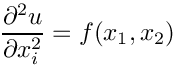such that the exact solution of the problem is given by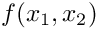which approaches a step function, oriented at an angle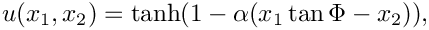within the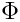plane, as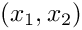becomes large. The figure below shows contour plots of the solution for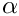for various angles. It illustrates how the mesh adaptation adjusts the mesh such the smallest elements are located in the region where the solution undergoes rapid change.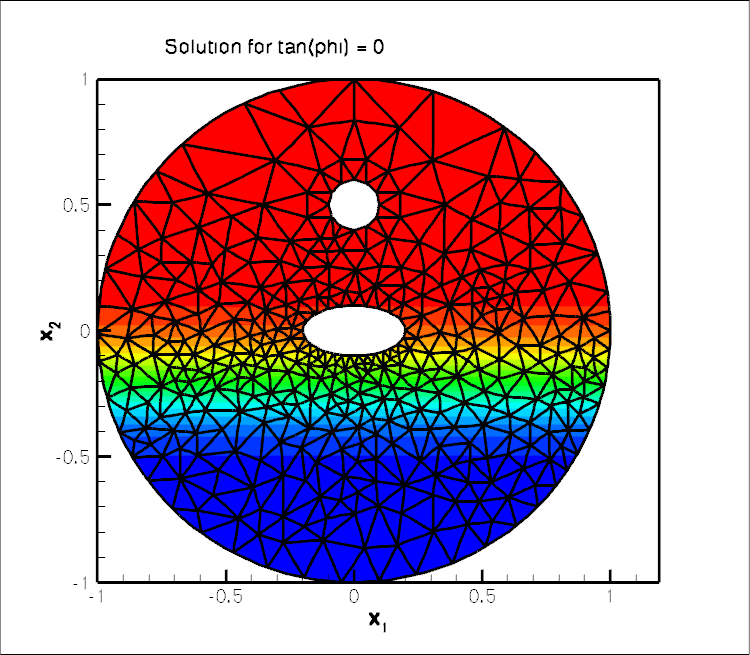Contour plot of the solution.

# Global parameters and functions

Following our usual practice, we use a namespace to define the source function and the exact solution.

//===== start_of_namespace=============================================
/// Namespace for exact solution for Poisson equation with "sharp step"
//=====================================================================
{
/// Parameter for steepness of "step"
double Alpha=5.0;
/// Parameter for angle Phi of "step"
double TanPhi=0.0;
/// Exact solution as a Vector
void get_exact_u(const Vector<double>& x, Vector<double>& u)
{
u=tanh(1.0-Alpha*(TanPhi*x-x));
}
/// Source function required to make the solution above an exact solution
void get_source(const Vector<double>& x, double& source)
{
source = 2.0*tanh(-1.0+Alpha*(TanPhi*x-x))*
(1.0-pow(tanh(-1.0+Alpha*(TanPhi*x-x)),2.0))*
Alpha*Alpha*TanPhi*TanPhi+2.0*tanh(-1.0+Alpha*(TanPhi*x-x))*
(1.0-pow(tanh(-1.0+Alpha*(TanPhi*x-x)),2.0))*Alpha*Alpha;
}
/// Zero function -- used to compute norm of the computed solution by
/// computing the norm of the error when compared against this.
void zero(const Vector<double>& x, Vector<double>& u)
{
u=0.0;
}
} // end of namespace
Namespace for exact solution for Poisson equation with "sharp step".
void zero(const Vector< double > &x, Vector< double > &u)
Zero function – used to compute norm of the computed solution by computing the norm of the error when...
double TanPhi
Parameter for angle Phi of "step".
void get_source(const Vector< double > &x, double &source)
Source function required to make the solution above an exact solution.
double Alpha
Parameter for steepness of "step".
void get_exact_u(const Vector< double > &x, Vector< double > &u)
Exact solution as a Vector.

# The driver code

We start by processing command line arguments which allow us to run the code in self-test mode and build the problem with "projectable" six-noded triangular Poisson elements.

//=======start_of_main========================================
/// Driver code for demo of inline triangle mesh generation
//============================================================
int main(int argc, char **argv)
{
// Store command line arguments
CommandLineArgs::setup(argc,argv);
// Define possible command line arguments and parse the ones that
// were actually specified
// Validation?
CommandLineArgs::specify_command_line_flag("--validation");
// Parse command line
CommandLineArgs::parse_and_assign();
// Doc what has actually been specified on the command line
CommandLineArgs::doc_specified_flags();
// Create problem
problem;
//////////////////////////////////////////////////////// ////////////////////////////////////////////...
int main(int argc, char **argv)
Driver code for demo of inline triangle mesh generation.

We then perform a parameter study, solving the problem for various orientations of the "step" and allowing a certain number of spatial adaptations per solve. (If the code is run in self-test mode, we perform fewer steps and allow for less adaptation to speed up the computation.)

// Loop over orientation of step
//==============================
unsigned nstep=5;
if (CommandLineArgs::command_line_flag_has_been_set("--validation"))
{
nstep=2;
}
for (unsigned i=0;i<nstep;i++)
{
//==============================
if (CommandLineArgs::command_line_flag_has_been_set("--validation"))
{
}
// Doc the solution
//=================
std::stringstream comment_stream;
comment_stream << "Solution for tan(phi) = " << TanhSolnForPoisson::TanPhi;
problem.doc_solution(comment_stream.str());
// Rotate orientation of solution
}
} //End of main
void doc_solution(const std::string &comment="")
Doc the solution.

# The problem class

The problem class contains the usual member functions. As discussed above, the boundary conditions and the source function have to be re-specified after every mesh adaptation since the adapted mesh contains completely new elements. This is done in the function complete_problem_setup(), discussed below, which is called from the Problem constructor and from actions_after_adapt(). We re-assign the Dirichlet boundary conditions in actions_before_newton_solve(), using a second helper function apply_boundary_conditions():

//==start_of_problem_class============================================
/// Class definition
//====================================================================
template<class ELEMENT>
class UnstructuredPoissonProblem : public virtual Problem
{
public:
/// Constructor
/// Destructor
/// Setup the problem again -- remember that the mesh has been
/// completely rebuilt and its element's don't have any
/// pointers to source fcts etc. yet
{
}
/// Update after solve (empty)
/// Update the problem specs before solve: Re-apply boundary conditons
{
}
/// Doc the solution
void doc_solution(const std::string& comment="");
private:
/// Doc info object for labeling output
DocInfo Doc_info;
/// Helper function to apply boundary conditions
/// Helper function to (re-)set boundary condition
/// and complete the build of all elements
/// Pointers to specific mesh
RefineableTriangleMesh<ELEMENT>* My_mesh_pt;
/// Trace file to document norm of solution
ofstream Trace_file;
}; // end_of_problem_class
void actions_before_newton_solve()
Update the problem specs before solve: Re-apply boundary conditons.
DocInfo Doc_info
Doc info object for labeling output.
void complete_problem_setup()
Helper function to (re-)set boundary condition and complete the build of all elements.
Actions after adapt: Setup the problem again – remember that the mesh has been completely rebuilt and...
void actions_after_newton_solve()
Update after solve (empty)
ofstream Trace_file
Trace file to document norm of solution.
void apply_boundary_conditions()
Helper function to apply boundary conditions.
RefineableTriangleMesh< ELEMENT > * My_mesh_pt
Pointers to specific mesh.

# The Problem constructor

Most of the problem constructor is concerned with the specification of the mesh boundaries. We start by generating a GeomObject that describes the circular outer boundary of the domain.

//==start_constructor=====================================================
/// Constructor
//========================================================================
template<class ELEMENT>
{
// Intrinsic coordinate along GeomObject
Vector<double> zeta(1);
// Position vector on GeomObject
Vector<double> posn(2);
// Ellipse defining the outer boundary
double x_center = 0.0;
double y_center = 0.0;
double A = 1.0;
double B = 1.0;
Ellipse * outer_boundary_ellipse_pt = new Ellipse(A,B);

This GeomObject is now used to describe the outer boundary in terms of a TriangleMeshClosedCurve object, a base class which can represent polygonal and curvilinear boundaries. We start by providing a pointer to this (yet-to-be-built) object.

// Pointer to the closed curve that defines the outer boundary
TriangleMeshClosedCurve* closed_curve_pt=0;

As discussed above, the closed outer boundary must be broken up into (at least) two distinct sub-boundaries to allow oomph-lib to automatically refine and setup boundary coordinates. We therefore create two TriangleMeshCurviLines, specifying

• the GeomObject that provides the exact curvilinear representation of the boundary,

• the start and end coordinates of the boundary on that GeomObject,

• the number of straight-line segments used to represent this boundary during the initial phase of the mesh generation process. Recall that nodes on this boundary are "snapped" onto the exact curvilinear boundary after the initial mesh is generated – the number of segments should therefore be sufficiently large to ensure that the "snapping" does not distort the elements next to the boundary too much. See How many vertices should I use to sample my curvilinear boundary? for a more detailed discussion of this issue.
// Provide storage for pointers to the two parts of the curvilinear boundary
Vector<TriangleMeshCurveSection*> outer_curvilinear_boundary_pt(2);

We choose five boundary segments for the first TriangleMeshCurviLine which represents the upper half of the boundary which we label as boundary 0,

// First bit
//----------
double zeta_start=0.0;
double zeta_end=MathematicalConstants::Pi;
unsigned nsegment=5;
unsigned boundary_id=0;
outer_curvilinear_boundary_pt=new TriangleMeshCurviLine(
outer_boundary_ellipse_pt,zeta_start,zeta_end,nsegment,boundary_id);

and eight segments for the lower half which we label as boundary 1:

// Second bit
//-----------
zeta_start=MathematicalConstants::Pi;
zeta_end=2.0*MathematicalConstants::Pi;
nsegment=8;
boundary_id=1;
outer_curvilinear_boundary_pt=new TriangleMeshCurviLine(
outer_boundary_ellipse_pt,zeta_start,zeta_end,nsegment,boundary_id);

We then combine the two TriangleMeshCurviLines to a TriangleMeshClosedCurve which describes the outer boundary.

// Combine to curvilinear boundary and define the
//--------------------------------
// outer boundary
//--------------------------------
closed_curve_pt=
new TriangleMeshClosedCurve(outer_curvilinear_boundary_pt);

Next we deal with the two inner (hole) boundaries

// Now build the holes
//====================
Vector<TriangleMeshClosedCurve*> hole_pt(2);

The first hole is a polygon whose 12 vertices we distribute along a circle of radius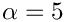, centred at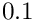As above, we break the closed boundary into two distinct sub-boundaries – this time represented by TriangleMeshPolyLines:

// Build polygonal hole
//=====================
// Build first hole: A circle
x_center = 0.0;
y_center = 0.5;
A = 0.1;
B = 0.1;
Ellipse* polygon_ellipse_pt=new Ellipse(A,B);
// Number of segments defining upper and lower half of the hole
unsigned n_seg = 6;
double unit_zeta = MathematicalConstants::Pi/double(n_seg);
// This hole is bounded by two distinct boundaries, each
// represented by its own polyline
Vector<TriangleMeshCurveSection*> hole_polyline_pt(2);

We create the vertex coordinates for the upper half of the polygonal hole,

// First boundary of polygonal hole
//---------------------------------
// Vertex coordinates
Vector<Vector<double> > bound_hole(n_seg+1);
for(unsigned ipoint=0; ipoint<n_seg+1;ipoint++)
{
// Resize the vector
bound_hole[ipoint].resize(2);
// Get the coordinates
zeta=unit_zeta*double(ipoint);
polygon_ellipse_pt->position(zeta,posn);
bound_hole[ipoint]=posn+x_center;
bound_hole[ipoint]=posn+y_center;
}

and build the TriangleMeshPolyLine, specifying a boundary ID:

// Specify the hole boundary id
unsigned boundary_id=2;
// Build the 1st hole polyline
hole_polyline_pt = new TriangleMeshPolyLine(bound_hole,boundary_id);

We repeat the exercise for the lower half which we turn into boundary 4:

// Second boundary of polygonal hole
//----------------------------------
for(unsigned ipoint=0; ipoint<n_seg+1;ipoint++)
{
// Resize the vector
bound_hole[ipoint].resize(2);
// Get the coordinates
zeta=(unit_zeta*double(ipoint))+MathematicalConstants::Pi;
polygon_ellipse_pt->position(zeta,posn);
bound_hole[ipoint]=posn+x_center;
bound_hole[ipoint]=posn+y_center;
}
// Specify the hole boundary id
boundary_id=3;
// Build the 2nd hole polyline
hole_polyline_pt = new TriangleMeshPolyLine(bound_hole,boundary_id);

Finally, we build the polygonal hole itself, specifying its constituent TriangleMeshPolyLines and the coordinate of a point inside the hole, which is required by Triangle:

// Build the polygonal hole
//-------------------------
// Inner hole center coordinates
Vector<double> hole_center(2);
hole_center=x_center;
hole_center=y_center;
hole_pt = new TriangleMeshPolygon(hole_polyline_pt, hole_center);

The construction of the second, curvilinear internal boundary (an ellipse centred at the origin) is virtually identical to the steps taken for the construction of the outer boundary, apart from the fact that, as an internal boundary, it again requires the specification of a point inside the hole.

// Build curvilinear hole
//======================
// Build second hole: Another ellipse
A = 0.2;
B = 0.1;
Ellipse* ellipse_pt=new Ellipse(A,B);
// Build the two parts of the curvilinear boundary
Vector<TriangleMeshCurveSection*> curvilinear_boundary_pt(2);
// First part of curvilinear boundary
//-----------------------------------
double zeta_start=0.0;
double zeta_end=MathematicalConstants::Pi;
unsigned nsegment=10;
boundary_id=4;
curvilinear_boundary_pt=new TriangleMeshCurviLine(
ellipse_pt,zeta_start,zeta_end,
nsegment,boundary_id);
// Second part of curvilinear boundary
//-------------------------------------
zeta_start=MathematicalConstants::Pi;
zeta_end=2.0*MathematicalConstants::Pi;
nsegment=15;
boundary_id=5;
curvilinear_boundary_pt=new TriangleMeshCurviLine(
ellipse_pt,zeta_start,zeta_end,
nsegment,boundary_id);
// Combine to hole
//----------------
Vector<double> hole_coords(2);
hole_coords=0.0;
hole_coords=0.0;
Vector<TriangleMeshClosedCurve*> curvilinear_hole_pt(1);
hole_pt=
new TriangleMeshClosedCurve(curvilinear_boundary_pt,
hole_coords);

## Construct the mesh

To facilitate the construction of the mesh TriangleMesh object we use the object TriangleMeshParameters. The only necessary argument for creating this object is the outer boundary. The definition of holes, internal boundaries and regions is explained in another tutorial. The object can also be used to control whether additional refinement may be performed on the mesh boundaries. The default behaviour is that such refinement will occur so that the highest quality mesh is obtained. In some cases, e.g. periodic boundary conditions, you may wish to ensure that each input boundary segment corresponds to a single element edge in the final mesh. This can be achieved for the outer boundary by calling TriangleMeshParameters::disable_boundary_refinement(). However, Triangle will still add additional points to any internal boundaries unless the additional function TriangleMeshParameters::disable_internal_boundary_refinement() is also called. The functions TriangleMeshParameters::enable_boundary_refinement() and TriangleMeshParameters::enable_internal_boundary_refinement() can be used to return to the default behaviour. Note that it is not currently possible to suppress refinement on the internal boundaries, but refine the outer boundary.

We can specify a target for the element sizes and pass it to the TriangleMeshParameters object as showed next:

// Now build the mesh
//===================
// Use the TriangleMeshParameters object for helping on the manage of the
// TriangleMesh parameters
TriangleMeshParameters triangle_mesh_parameters(closed_curve_pt);
// Specify the closed curve using the TriangleMeshParameters object
triangle_mesh_parameters.internal_closed_curve_pt() = hole_pt;
// Specify the maximum area element
double uniform_element_area=0.2;
triangle_mesh_parameters.element_area() = uniform_element_area;
// Create the mesh
My_mesh_pt=new
RefineableTriangleMesh<ELEMENT>(triangle_mesh_parameters);
// Store as the problem's one and only mesh
Problem::mesh_pt()=My_mesh_pt;

We specify a spatial error estimator and limit the maximum and minimum element sizes,

// Set error estimator for bulk mesh
Z2ErrorEstimator* error_estimator_pt=new Z2ErrorEstimator;
My_mesh_pt->spatial_error_estimator_pt()=error_estimator_pt;
// Set element size limits
My_mesh_pt->max_element_size()=0.2;
My_mesh_pt->min_element_size()=0.002;

before completing the problem setup (see below) and assigning the equation numbers.

// Set boundary condition and complete the build of all elements
complete_problem_setup();
// Open trace file
char filename;
sprintf(filename,"RESLT/trace.dat");
Trace_file.open(filename);
// Setup equation numbering scheme
oomph_info <<"Number of equations: "
<< this->assign_eqn_numbers() << std::endl;
} // end_of_constructor

# Completing the problem setup

As discussed above, the helper function complete_problem_setup() starts by (re-)applying the boundary conditions by pinning the nodal values on all mesh boundaries,

//==start_of_complete======================================================
/// Set boundary condition exactly, and complete the build of
/// all elements
//========================================================================
template<class ELEMENT>
{
// Set the boundary conditions for problem: All nodes are
// free by default -- just pin the ones that have Dirichlet conditions
// here.
unsigned nbound=My_mesh_pt->nboundary();
for(unsigned ibound=0;ibound<nbound;ibound++)
{
unsigned num_nod=My_mesh_pt->nboundary_node(ibound);
for (unsigned inod=0;inod<num_nod;inod++)
{
// Get node
Node* nod_pt=My_mesh_pt->boundary_node_pt(ibound,inod);
// Pin one-and-only unknown value
nod_pt->pin(0);
}
} // end loop over boundaries

specifies the source function pointer for all elements,

// Complete the build of all elements so they are fully functional
unsigned n_element = My_mesh_pt->nelement();
for(unsigned e=0;e<n_element;e++)
{
// Upcast from GeneralisedElement to the present element
ELEMENT* el_pt = dynamic_cast<ELEMENT*>(My_mesh_pt->element_pt(e));
//Set the source function pointer
el_pt->source_fct_pt() = &TanhSolnForPoisson::get_source;
}

and then re-sets the boundary values:

// Re-apply Dirichlet boundary conditions (projection ignores
// boundary conditions!)
apply_boundary_conditions();
}

# Assigning the boundary values

The function apply_boundary_conditions() does exactly what is says: It loops over all boundary nodes and assigns the value according the exact solution specified in the namespace TanhSolnForPoisson.

//==start_of_apply_bc=====================================================
/// Helper function to apply boundary conditions
//========================================================================
template<class ELEMENT>
{
// Loop over all boundary nodes
unsigned nbound=this->My_mesh_pt->nboundary();
for(unsigned ibound=0;ibound<nbound;ibound++)
{
unsigned num_nod=this->My_mesh_pt->nboundary_node(ibound);
for (unsigned inod=0;inod<num_nod;inod++)
{
// Get node
Node* nod_pt=this->My_mesh_pt->boundary_node_pt(ibound,inod);
// Extract nodal coordinates from node:
Vector<double> x(2);
x=nod_pt->x(0);
x=nod_pt->x(1);
// Compute the value of the exact solution at the nodal point
Vector<double> u(1);
// Assign the value to the one (and only) nodal value at this node
nod_pt->set_value(0,u);
}
}
} // end set bc

# Post-processing

We compare the computed solution against the exact solution:

//==start_of_doc_solution=================================================
/// Doc the solution
//========================================================================
template<class ELEMENT>
std::string& comment)
{
ofstream some_file;
char filename;
// Number of plot points
unsigned npts;
npts=5;
sprintf(filename,"RESLT/soln%i.dat",Doc_info.number());
some_file.open(filename);
this->My_mesh_pt->output(some_file,npts);
some_file << "TEXT X = 22, Y = 92, CS=FRAME T = \""
<< comment << "\"\n";
some_file.close();
// Output exact solution
//----------------------
sprintf(filename,"RESLT/exact_soln%i.dat",Doc_info.number());
some_file.open(filename);
My_mesh_pt->output_fct(some_file,npts,TanhSolnForPoisson::get_exact_u);
some_file.close();
// Output boundaries
//------------------
sprintf(filename,"RESLT/boundaries%i.dat",Doc_info.number());
some_file.open(filename);
My_mesh_pt->output_boundaries(some_file);
some_file.close();
// Doc error and return of the square of the L2 error
//---------------------------------------------------
double error,norm,dummy_error,zero_norm;
sprintf(filename,"RESLT/error%i.dat",Doc_info.number());
some_file.open(filename);
My_mesh_pt->compute_error(some_file,TanhSolnForPoisson::get_exact_u,
error,norm);
My_mesh_pt->compute_error(some_file,TanhSolnForPoisson::zero,
dummy_error,zero_norm);
some_file.close();
// Doc L2 error and norm of solution
oomph_info << "\nNorm of error : " << sqrt(error) << std::endl;
oomph_info << "Norm of exact solution: " << sqrt(norm) << std::endl;
oomph_info << "Norm of computed solution: " << sqrt(dummy_error) << std::endl;
Trace_file << sqrt(norm) << " " << sqrt(dummy_error) << std::endl;
// Increment the doc_info number
Doc_info.number()++;
} // end of doc

## Upgrading elements to become "projectable"

As discussed above, a key step in the "mesh-adaptation-by-mesh-regeneration" procedure is the projection of the solution from the old to the new mesh. The ability to perform this projection fully-automatically during the mesh adaptation requires the elements to be "wrapped" in the templated ProjectableElement<ELEMENT> class. This class is derived from the ProjectableElementBase base class which specifies a number of pure virtual functions that must be specified for each specific element type. For the Poisson problem considered here these functions are already provided in the ProjectablePoissonElement<ELEMENT> class. Similar wrappers exist for many other equations. If you want to "upgrade" your own elements to become projectable, inspect the prototypes for the relevant pure virtual functions which are defined in

src/generic/projection.h

while the specific implementation for the projectable Poisson elements is provided in

src/poisson/poisson_elements.h

Of course, you can avoid the additional work by dispensing with adaptivity and simply generating a sufficiently fine (uniform) mesh. This is illustrated in the alternative driver code

## How many vertices should I use to sample my curvilinear boundary?

As discussed above, meshes with curvilinear boundaries are created in a two-stage process. Initially, Triangle generates a mesh with polygonal boundaries using a user-specified number of vertices that are evenly distributed along the relevant GeomObject. The nodes on that boundary are then "snapped" onto the actual curvilinear boundary in a post-processing step. The decision of how many vertices to choose involves a compromise between two conflicting demands:

• The number of boundary nodes created by Triangle will be at least as big as the number of vertices specified. Triangle may add additional boundary nodes to generate a mesh of sufficient quality but it will not remove any vertices. Using a very large number of vertices can therefore lead to unnecessarily fine meshes.

• If the number of vertices is too small, the polygonal representation of the domain boundary may be a poor approximation to the actual curvilinear boundary. Elements near such boundaries may become (too) strongly distorted when nodes are "snapped" onto the curvilinear boundary. This often manifests itself in inverted elements. (An element is considered inverted if the Jacobian of the mapping between local and global coordinates becomes non-positive anywhere. Note that negative Jacobians may occur in the interior of elements (e.g. at their Gauss points) even if a plot of the element, based on its nodal positions, still looks "OK").

## Exercises

1. Change the outer curvilinear boundary to a polygonal boundary (you can cheat – the relevant code is already contained in the driver code but it's "hidden" with ifdefs; this code also documents the re-distribution of straight-line segments between different polylines, a capability that is important in certain free-boundary problems).

2. Vary the number of vertices used for the initial polygonal representation of the curvilinear hole to establish what number is required to avoid the inversion of elements during the "snap-nodes-to-the-curvilinear-boundary" phase.

3. Create "projectable" advection diffusion elements to solve the the advection diffusion problem discussed in another tutorial, using spatial adaptation on an unstructured mesh.

# Appendix A: Generalization for polylines and curvilines

The objects, TriangleMeshPolyLine and TriangleMeshCurviLine inherit the properties of a more general representation called TriangleMeshCurveSection. This allows one to define more general boundaries as a combination of TriangleMeshPolyLines and TriangleMeshCurviLines. Therefore, if we want to define a more general closed curve use the TriangleMeshClosedCurve object. You may notice the use of this object through the example code when defining the outer boundary.

For the interested reader, the class diagram showing the hierarchy of the mentioned objects is showed on the next figure.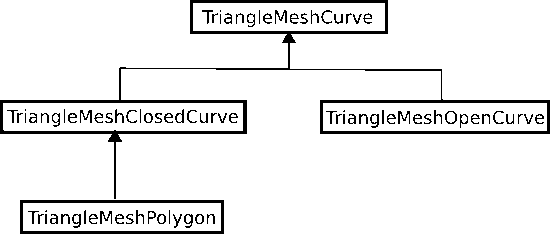The hierarchy of the TriangleMesh objects.

Note the TriangleMeshOpenCurve object, which allows to define internal boundaries on the domain, explained on another tutorial